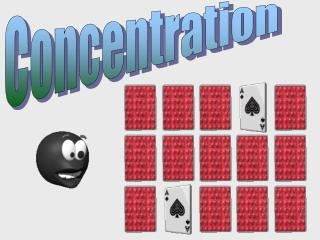DownloadDownload PresentationConcentration

# Concentration

Télécharger la présentation## Concentration

- - - - - - - - - - - - - - - - - - - - - - - - - - - E N D - - - - - - - - - - - - - - - - - - - - - - - - - - -
##### Presentation Transcript

1. Concentration

2. Solution Concentration Read 281 – 283. Try questions 1 – 8 (show work) Concentration = quantity of solute quantity of solution (not solvent) There are 3 basic ways to express concentration: 1) percentages, 2) very low concentrations, and 3) molar concentrations 1) % concentration can be in V/V, W/W, or W/V • Like most %s, V/V and W/W need to have the same units on top and bottom. • W/V is sort of in the same units; V is mostly water and water’s density is 1 g/mL or 1 kg/L 3gH2O2/100mLsolution  3gH2O2/100gsolution

3. Solution Concentration 2) Expressing concentrations in parts per million (ppm) requires the unit on top to be 1,000,000 times smaller than the unit on the bottom E.g. 1 mg/kg or g/g • Multiples of 1000 are expressed in this order _, m_, _, k_ (“_” is the base unit) (pg.631) • Notice that any units expressed as a volume must be referring to a water solution (1L = 1kg) • For parts per billion (ppb), the top unit would have to be 1,000,000,000 times smaller 3) Molar concentration is the most commonly used in chemistry. Ensure that units are mol/L.

4. Percentage concentration (V/V, W/V, W/W), very low concentration, molar concentration • % V/V = 4.1 L / 55 L = 7.5% V/V • % W/V = 16 g / 50 mL = 32% W/V • % W/W = 1.7 g / 35.0 g = 4.9% W/W • 8 ppm = 8 _ / 1 L, the units should be 1 million times smaller than 1L (or 1kg): 8ppm = 8 mg/L so the mass in 1 L is 8 mg. • 3.2 mg/0.59 kg = 5.424 mg/kg = 5.4 ppm • a) ppb is 1000 times smaller than ppm b) 1 g/109 mL, 1 mg/1000 L, 1 g/L, 1 mg/kL, 1 mg/Mg, 1 g/kg • 0.11 mol / 0.060 L = 1.8 mol/L

5. More practice 10 g / 260 g = 3.8 % W/W 30 mL / 280 mL = 11% V/V (in reality may be off) 8.0 g / 100 g = 8% W/W • What is the % W/W of copper in an alloy when 10 g of Cu is mixed with 250 g of Zn? • What is approximate % V/V if 30 mL of pure ethanol is added to 250 mL of water? • What is the %W/W if 8.0g copper is added to enough zinc to produce 100 g of an alloy? Read 284 – 287. Do Q 11 – 17 (show work)

6. Answers • 500 mL x 70 mL/100 mL = 350 mL • 250 mL x 1000 = 250 L 250 L x 3 kg/100 L = 7.5 kg • 1.5 ppm = 1.5 mg/L 0.250 L x 1.5 mg/L = 0.38 mg • 75 L x 0.055 mol/L = 4.1 mol • 0.050 L x 5.0 mol/L = 0.25 mol • 0.500 mol x 1 L/1.24 mol = 0.403L or 403mL • 0.14 mol x 1 L/2.6 mol = 0.0538 L = 54 mL For more lessons, visit www.chalkbored.com